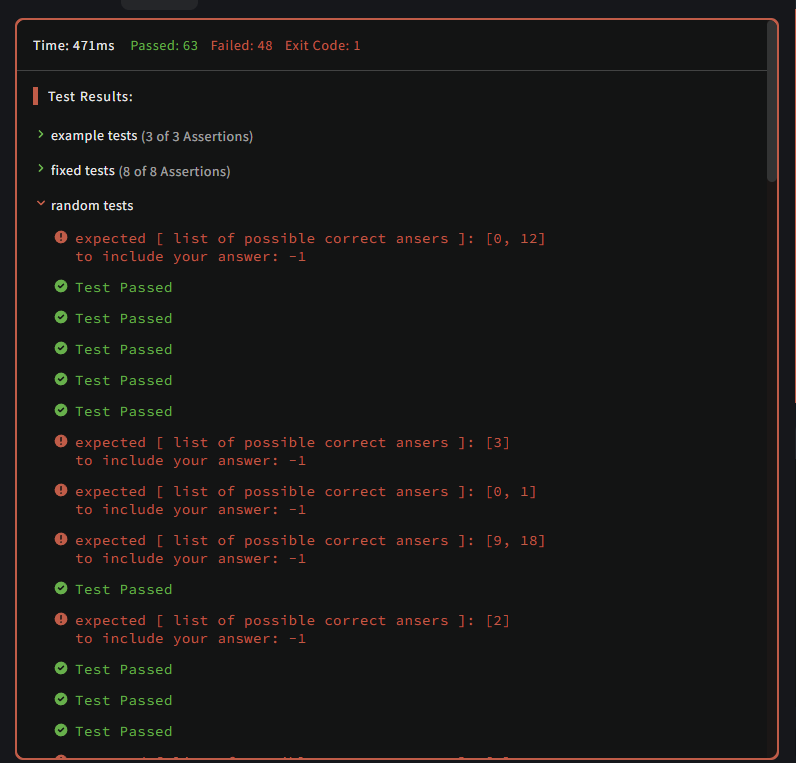# 【每日一题20220509】刚好大你‍ 给定一个数字列表(无重复)和一个索引值，请编写一个函数，找出刚好比给定索引对应的数字值大一丢丢的数字。 如果存在则返回该数字，如果不存在则返回-1。

【示例】

``````def solution(nums: list, idx: int)-> int:

assert solution([4, 1, 3, 5, 6], 0) == 3
assert solution([4, 1, 3, 5, 6], 4) == -1
assert solution([1, 3, 5, 2, 4], 0) == 3
``````
``````def solution(nums:list,idx:int)-> int:
big_nums = [nums[i] for i in range(len(nums)) if nums[i]>nums[idx]]
if len(big_nums)>0:
return nums.index(min(big_nums))
else:
return -1
if __name__ == '__main__':
print(solution([4, 1, 3, 5, 6], 0))
print(solution([4, 1, 3, 5, 6], 4))
print(solution([1, 3, 5, 2, 4], 0))
print(solution([1, 3, 5, 7], 0))

``````

“找出刚好比给定索引对应的数字值大一点的数字"中“大一点”有歧义``````def solution(nums: list, idx: int)-> int:
if (n := nums[idx] + 1) in nums:
return nums.index(n)
else:
return -1
````````````def solution(nums: list, idx: int) -> int:
li = []
for i in nums:
if i > nums[idx]:
li.append(i)
if len(li) == 0:
return -1
else:
return nums.index(min(li))

assert solution([4, 1, 3, 5, 6], 0) == 3
assert solution([4, 1, 3, 5, 6], 4) == -1
assert solution([1, 3, 5, 2, 4], 0) == 3
``````
``````
def solution(nums: list, idx: int) -> int:
ls2 = [i for i in nums if i > nums[idx]]
if len(ls2) > 0:
return nums.index(min(ls2))
else:
return -1

if __name__ == '__main__':
assert solution([4, 1, 3, 5, 6], 0) == 3
assert solution([4, 1, 3, 5, 6], 4) == -1
assert solution([1, 3, 5, 2, 4], 0) == 3
``````
``````def solution(nums: list, idx: int)-> int:
return -1 if sorted(nums[:]).index(nums[idx]) == len(sorted(nums[:])) - 1 \
else nums.index(sorted(nums[:])[sorted(nums[:]).index(nums[idx]) + 1])

assert solution([4, 1, 3, 5, 6], 0) == 3
assert solution([4, 1, 3, 5, 6], 4) == -1
assert solution([1, 3, 5, 2, 4], 0) == 3
``````
``````# 由于题目及示例表述问题，此处按照返回索引处理
def solution(nums: list, idx: int)-> int:
return nums.index(sorted(nums)[sorted(nums).index(nums[idx])+1]) if sorted(nums).index(nums[idx])+1 < len(nums) else -1

assert solution([4, 1, 3, 5, 6], 0) == 3
assert solution([4, 1, 3, 5, 6], 4) == -1
assert solution([1, 3, 5, 2, 4], 0) == 3
``````

``````def solution(nums: list, idx: int)-> int:
if len(n := list(filter(lambda x: x > nums[idx], nums))) > 0:
return nums.index(min(n))
else:
return -1
``````

``````def solution(nums: list, idx: int) -> int:
nums_bak = [i for i in nums]
nums.sort()
data = nums_bak[idx]
if data == max(nums):
return -1
else:
i = nums.index(data)
result = nums[i+1]
return nums_bak.index(result)

assert solution([4, 1, 3, 5, 6], 0) == 3
assert solution([4, 1, 3, 5, 6], 4) == -1
assert solution([1, 3, 5, 2, 4], 0) == 3

``````

``````def solution(nums: list, idx: int)-> int:
data = nums[idx]
if max(nums)==data:
return -1
return nums.index(min([num for num in nums if num > data]))

assert solution([4, 1, 3, 5, 6], 0) == 3
assert solution([4, 1, 3, 5, 6], 4) == -1
assert solution([1, 3, 5, 2, 4], 0) == 3
``````
``````def solution(nums: list, idx: int)-> int:
target = nums[idx]
litter_big = None
litter_big_index = -1
for index,value in enumerate(nums):
if value>target and (litter_big is None or value < litter_big):
litter_big = value
litter_big_index = index
return litter_big_index
``````